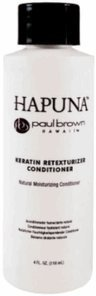# Paul Brown Hawaii Hapuna Texturizer Conditioner, 4 Oz.

• \$30.89
• Save \$2.66

• A

• n

• a

• t

• u

• r

• a

• l

• m

• o

• i

• s

• t

• u

• r

• i

• z

• i

• n

• g

• c

• o

• n

• d

• i

• t

• i

• o

• n

• e

• r

• F

• R

• E

• E

• o

• f

• p

• a

• r

• a

• b

• e

• n

• s

• a

• n

• d

• p

• e

• t

• r

• o

• c

• h

• e

• m

• i

• c

• a

• l

• s

• t

• h

• i

• s

• r

• i

• c

• h

• a

• n

• d

• h

• e

• a

• r

• t

• y

• c

• o

• n

• d

• i

• t

• i

• o

• n

• e

• r

• i

• s

• h

• i

• g

• h

• l

• y

• c

• o

• n

• c

• e

• n

• t

• r

• a

• t

• e

• d

• t

• o

• i

• n

• g

• e

• n

• i

• o

• u

• s

• l

• y

• m

• o

• i

• s

• t

• u

• r

• i

• z

• e

• s

• t

• r

• e

• n

• g

• t

• h

• e

• n

• r

• e

• p

• a

• i

• r

• a

• n

• d

• p

• r

• o

• t

• e

• c

• t

• t

• h

• e

• h

• a

• i

• r

• a

• g

• a

• i

• n

• s

• t

• b

• r

• e

• a

• k

• a

• g

• e

• a

• n

• d

• d

• a

• m

• a

• g

• e

• .

• Size : 4 oz.

Free of parabens and petrochemicals, this rich and luxurious conditioner is highly concentrated to ingeniously moisturize, strengthen, repair and protect the hair against breakage and damage. By conditioning and fortifying each and every hair strand with aloe vera, panthenol, soy protein and essential hawaiian oils, this conditioner enhances the process of the Hapuna Keratin Retexturizer and brings the hair into a healthy, more manageable state.

every pages